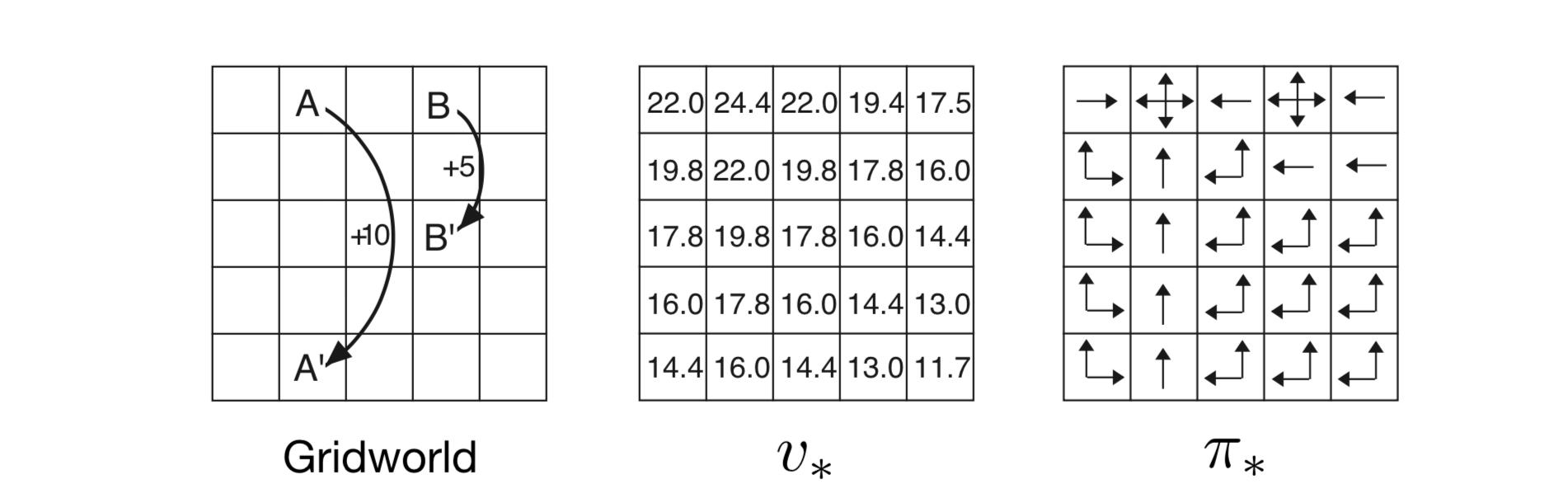# 第3章 有限马尔可夫决策过程¶

MDP是强化学习问题的数学理想化形式，可以对其进行精确的理论陈述。 我们介绍问题数学结构的关键元素，如回归，值函数和Bellman方程。 我们试图传达可能被用作有限MDP的各种可能的应用程序。 与所有人工智能一样，在适用范围和数学易处理性之间存在着一种矛盾。 在本章中，我们将介绍这种矛盾关系，并讨论它所暗示的一些权衡和挑战。 第17章介绍了在MDP之外进行强化学习的一些方法。

## 3.1 个体环境接口¶

MDP旨在直接构建从交互中学习以实现目标的问题。 学习者和决策者被称为 个体（agent）。它与之交互的东西，包括个体之外的所有东西，被称为 环境。 这些交互持续不断，个体选择动作同时环境响应那些动作并向个体呈现新情况 。 环境还产生奖励，这是个体通过其行动选择寻求最大化的特殊数值。(1)$S_0,A_0,R_1,S_1,A_1,R_2,S_2,A_2,R_3,\dots$

(2)$p(s^\prime,r|s,a) \doteq Pr\{S_t=s^\prime,R_t=r|S_{t-1}=s,A_{t-1}=a\}$

(3)$\sum_{s^\prime \in \mathcal{S}}\sum_{r \in \mathcal{R}}p(s^\prime,r|s,a)=1，对所有 s \in \mathcal{S}，a \in \mathcal{A}(s)$

(4)$p(s^\prime|s,a) \doteq Pr\{S_t=s^\prime|S_{t-1}=s,A_{t-1}=a\}=\sum_{r\in\mathcal{R}}p(s^\prime,r|s,a)$

(5)$r(s,a)\doteq\mathbb{E}\left[R_t|S_{t-1}=s,A_{t-1}=a\right]=\sum_{r\in\mathcal{R}}r\sum_{s^\prime\in\mathcal{S}}p(s^\prime,r|s,a)$

(6)$r(s,a,s^\prime)\doteq\mathbb{E}\left[R_t|S_{t-1}=s,A_{t-1}=a,S_t=s^\prime\right]=\sum_{r\in\mathcal{R}}r\frac{p(s^\prime,r|s,a)}{p(s^\prime|s,a)}$

MDP框架是抽象和灵活的，可以以不同的方式应用在很多不同的问题上。 例如，时间步长不需要指固定的实时间隔；它们可以指任意连续的决策和行动阶段。 这些动作可以是低级控制，例如施加到机器人手臂的电动机的电压，或高级​​决策，例如是否要吃午餐或进入研究生院。 同样，状态也可以采取各种各样的形式。它们可以完全由低级感觉决定，例如直接传感器读数， 或者它们可以更高级和抽象，例如房间中物体的符号描述。 可以基于对过去的感觉的记忆，甚至是完全精神的或主观的来构成一个状态。 例如，个体可能处于不确定对象在哪里的状态，或者在某些明确定义的意义上感到惊讶的状态。 同样，某些行为可能完全是精神上的或可计算的。例如，某些操作可能会控制代理选择考虑的内容，或者它关注的重点。 一般而言，行动可以是我们想要学习如何制定的任何决定，而状态可以是我们可以知道的任何可能有助于制作它们的任何事物。

MDP框架是从相互作用的目标导向学习的问题中抽象出来的。 它提出无论传感，记忆和控制装置的细节，以及任何目标试图达到的目标， 学习目标导向行为的任何问题都可以减少为个体及其环境之间来回传递的三个信号： 一个信号表示个体做出的选择（动作），一个信号表示作出选择的基础（状态），以及另一个信号来定义个体的目标（奖励）。 这个框架可能不足以有效地代表所有决策学习问题，但它已被证明是广泛有用和适用的。## 3.3 回报和情节¶

(7)$G_{t} \doteq R_{t+1} +R_{t+2} + R_{t+3} + \dots + R_{T}，$

(8)$G_{t} \doteq R_{t+1} + \gamma R_{t+2} + \gamma^2 R_{t+3} + \dots = \sum_{k=0}^{\infty}\gamma^k R_{t+k+1}$

(9)\begin{split}\begin{aligned} G_{t} &\doteq R_{t+1} + \gamma R_{t+2} + \gamma^2 R_{t+3} + \gamma^3 R_{t+4} + \dots \\ &= R_{t+1} + \gamma(R_{t+2} + \gamma R_{t+3} + \gamma^2 R_{t+4} + \dots) \\ &= R_{t+1} + \gamma G_{t+1} \end{aligned}\end{split}

(10)$G_t = \sum_{k=0}^{\infty}\gamma^k = \frac{1}{1-\gamma}$## 3.4 情节和持续任务的统一符号¶state transition diagram

(11)$G_t \doteq \sum_{k=t+1}^{T} \gamma^{k-t-1} R_k$

## 3.5 策略和价值函数¶

(12)$v_\pi(s) \doteq \mathbb{E}_\pi\left[G_t|S_t=s\right] = \mathbb{E}_\pi\left[\sum_{k=0}^{\infty} \gamma^k R_{t+k+1}|S_t=s\right]，对所有 s\in \mathbb{S}$

(13)$q_\pi(s,a) \doteq \mathbb{E}_\pi\left[G_t|S_t=s,A_t=a\right] = \mathbb{E}_\pi\left[\sum^{\infty}_{k=0}\gamma^kR_{t+k+1}|S_t=s,A_t=a\right]$

(14)\begin{split}\begin{aligned} v_\pi(s) &\doteq \mathbb{E}_\pi[G_t|S_t=s] \\ &= \mathbb{E}_\pi[R_{t+1} + \gamma G_{t+1}|S_t=s] (由 (3.9)) \\ &= \sum_a\pi(a|s) \sum_{s^\prime}\sum_r p(s^\prime,r|s,a) \left[r+\gamma\mathbb{E}_\pi[G_{t+1}|S_{t+1}=s^\prime]\right] \\ &= \sum_a\pi(a|s) \sum_{s^\prime,r}p(s^\prime,r|s,a)[r+\gamma v_\pi(s^\prime)], 对所有 s\in\mathcal{S} \end{aligned}\end{split}## 3.6 最优策略和最优价值函数¶

(15)$v_*(s) \doteq \max_\pi v_\pi(s)，$

(16)$q_*(s,a) \doteq \max_\pi q_\pi(s,a)$

(17)$q_*(s,a) = \mathbb{E}\left[R_{t+1}+\gamma v_* (S_{t+1})|S_t=s,A_t=a\right]$

\begin{split}\begin{aligned} v_*(s) &= \max_{a\in\mathcal{A}(s)} q_{\pi_*}(s,a) \\ &=\max_a \mathbb{E}_{\pi_*}[G_t|S_t=s,A_t=a] \\ &=\max_a \mathbb{E}_{\pi_*}[R_{t+1}+\gamma G_{t+1}|S_t=s,A_t=a] &(由(3.9)式) \\ &=\max_a \mathbb{E}[R_{t+1}+\gamma v_*(S_{t+1})|S_t=s,A_t=a] &(3.18) \\ &=\max_{a\in \mathcal{A}(s)}\sum_{s^\prime,r} p(s^\prime,r|s,a)[r+\gamma v_*(s^\prime)] &(3.19) \end{aligned}\end{split}

(18)\begin{split}\begin{aligned} q_*(s,a) &= \mathbb{E}\left[R_{t+1}+\gamma\sum_{a^\prime}q_*(S_{t+1,a^\prime})|S_t=s,A_t=a\right] \\ &=\sum_{s^\prime,r}p(s^\prime,r|s,a)[r+\gamma \max_{a^\prime}q_*(s^\prime,a^\prime)] \end{aligned}\end{split}$$q_*$$ 使选择最优动作更容易。 使用 $$q_*$$ ，个体甚至不需要进行一步一步的查询： 对于任何状态 $$s$$，它可以简单地发现任何使 $$q_*(s,a)$$ 最大化的动作。 动作价值函数有效地缓存了所有一步一步搜索的结果。 它提供最优的期望长期回报作为本地并立即可用于每个状态—动作对的值。 因此，代表状态-动作对的功能而不仅仅是状态的代价， 最优动作-价值函数允许选择最优动作而不必知道关于可能的后继状态及其值的任何信息，即，不必要了解环境的动态。\begin{split}\begin{aligned} v_*(h)&=\max\left\{ \begin{array}{lr} p(h|h,s)[r(h,s,h)+\gamma v_*(h)]+p(l|h,s)[r(h,s,l)+\gamma v_*(l)],\\ p(h|h,w)[r(h,w,h)+\gamma v_*(h)]+p(l|h,w)[r(h,w,l)+\gamma v_*(l)] \end{array}\right\} \\ &=\max\left\{ \begin{array}{lr} \alpha[r_s + \gamma v_*(h)]+(1-\alpha)[r_s +\gamma v_*(l)],\\ l[r_w+\gamma v_*(h)]+0[r_w+\gamma v_*(l)] \end{array}\right\} \\ &=\max\left\{ \begin{array}{lr} r_s+\gamma[\alpha v_*(h)+(1-\alpha)v_*(l)],\\ r_w + \gamma v_*(h) \end{array}\right\} \end{aligned}\end{split}

\begin{split}v_*(l)=\max\left\{ \begin{aligned} &\beta r_s - 3(1-\beta)+\gamma[(1-\beta)v_*(h)+\beta v_*(l)], \\ &r_w + \gamma v_*(l),\\ &\gamma v_*(h) \end{aligned} \right\}\end{split}

## 书目和历史评论¶

MDP理论通过例如Bertsekas（2005），White（1969），Whittle（1982，1983）和Puterman（1994）来处理。 Ross（1983）给出了对有限情况的特别紧凑的处理。MDP也在随机最优控制的标题下进行研究， 其中自适应最优控制方法与强化学习最密切相关（例如，Kumar，1985；Kumar和Varaiya，1986）。

MDP理论从一系列发展而来，以理解在不确定性下制定决策序列的问题，每个决策都取决于先前的决策及其结果。 它有时被称为多阶段决策过程或顺序决策过程的理论，并且源于关于顺序抽样的统计文献， 从Thompson（1933,1934）和Robbins（1952）的论文开始，我们在第2章中引用了有赌博机问题（如果制定为多情况问题，则是典型的MDP）。

3.1 我们用 $$p(s^\prime,r|s,a)$$ 表征MDP的动力学有点不寻常。 在MDP文献中更常见的是根据状态转移概率 $$p(s^\prime|s,a)$$ 和预期的下一个奖励 $$r(s,a)$$ 来描述动态。 然而，在强化学习中，我们更多时候必须参考个人的实际或样本奖励（而不仅仅是他们的期望值）。 我们的符号也使得 $$S_t$$$$R_t$$ 通常共同确定更加平稳，因此必须具有相同的时间索引。 在强化学习教学中，我们发现我们的概念在概念上更直接，更容易理解。

3.2 奖励假设由Michael Littman（个人通信）提出。

3.3-4 情节连续 任务的术语与MDP文献中常用的术语不同。 在该文献中，通常区分三种类型的任务：（1）有限时间任务，其中交互在特定固定数量的时间步骤之后终止； （2）无限期任务，其中交互可以持续任意长，但最终必须终止；（3）无限期任务，其中交互不会终止。 我们的情节和持续任务分别类似于不定限期和无限期任务，但我们更倾向于强调交互性质的差异。 这种差异似乎比通常术语强调的目标函数的差异更为重要。情节任务经常使用不定时间目标函数和持续任务无限期目标函数， 但我们认为这是一个常见的巧合，而不是根本的差异。

3.5-6 从长远来看，基于好的或坏的分配价值具有古老的根源。 在控制理论中，将状态映射到代表控制决策的长期结果的数值是最优控制理论的关键部分， 最优控制理论是在20世纪50年代通过扩展19世纪经典力学的状态函数理论而开发的（参见，例如，Schultz和Melsa，1967年）。 在描述计算机如何编程下棋时，Shannon（1950）考虑到国际象棋位置的长期优势和劣势，建议使用评估功能。

Watkins（1989）用于估计 $$q_*$$ 的Q学习算法（第6章）使得动作-价值函数成为强化学习的重要部分， 因此这些函数通常被称为“Q函数”。但是动作-价值函数的概念比这更古老了。 Shannon（1950）提出，国际象棋游戏程序可以使用函数 $$h(P,M)$$ 来确定位置 $$P$$ 中的移动 $$M$$ 是否值得探索。 Michie（1961,1963）的MENACE系统和Mi​​chie and Chambers（1968）的BOXES系统可以理解为估算行动价值函数。 在经典物理学中，汉密尔顿的主要功能是行动价值函数；关于这个函数，牛顿动力学是贪婪的（例如，Goldstein，1957）。 行动价值函数在Denardo（1967）关于收缩映射的动态规划理论处理中也发挥了核心作用。 贝尔曼最优性方程（对于 $$v_*$$）由Richard Bellman（1957a）推广，他将其称为“基本函数方程”。 连续时间和状态问题的贝尔曼最优方程的对应物被称为Hamilton-Jacobi-Bellman方程式（或通常只是Hamilton-Jacobiequation）， 表明其在经典物理学中的根源（例如，Schultz和Melsa，1967）。高尔夫的例子是克里斯沃特金斯建议的。

  我们使用术语个体，环境和动作，而不是工程师术语控制器，受控系统（或工厂）和控制信号，因为它们对更广泛的受众有意义。
  我们将注意力限制在离散时间以使事情尽可能简单，即使许多想法可以延伸到连续时间情况 （例如，参见Bertsekas和Tsitsiklis，1996；Werbos，1992；Doya，1996）。
  我们使用 $$R_{t+1}$$ 而不是 $$R_{t}$$ 来表示归因于 $$A_{t}$$ 的奖励， 因为它强调下一个奖励和下一个状态 $$R_{t+1}$$ 和 $$S_{t+1}$$ 共同确定。 不幸的是，这两种惯例在文献中都被广泛使用。
  更好的方式是传授这种先验知识是最初的策略或价值功能，或对这些的影响。 参见Lin（1992），Maclin和Shavlik（1994）和Clouse（1996）。
  传授这种先验知识的更好的地方是初始策略或初始价值功能，或影响这些。
  第17.4节进一步探讨了设计有效奖励信号的问题。
  情节有时在文献中被称为“试验”。